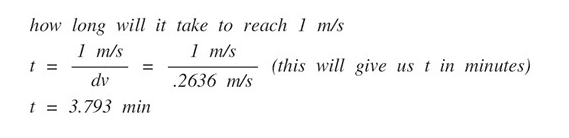# How to calculate time with delta-v and velocity?

• B
alberto91
Is this correct?Why the time is minutes instead of seconds?

Thanks!

•Delta2

Gold Member
By dimensional analysis your t has no units.

alberto91
By dimensional analysis your t has no units.

And how can I calculate that equation?

Gold Member
What I mean is you're dividing two expressions with m/s as units, which cancel out. This tells you you're doing something wrong somewhere. I guess you want to use t=d/v? Then d is given in meters ( or another unit of distance) . In your case you used 1 m/s , which is not a measure of distance.

alberto91
What I mean is you're dividing two expressions with m/s as units, which cancel out. This tells you you're doing something wrong somewhere. I guess you want to use t=d/v? Then d is given in meters ( or another unit of distance) . In your case you used 1 m/s , which is not a measure of distance.

I want to use delta-vee (dv)

mfig
To find the time you need a relationship between velocity and time such as the definition of acceleration:

##\frac{dv}{dt}=a##

Then separate and integrate:

##dv=adt \rightarrow \int_{0}^{v_{needed}} dv = \int_{0}^{t_{needed}}a dt##

This gives

##v_{needed} = at_{needed}##

So to find the time needed to get to the needed velocity, simply divide.

##t_{needed} = v_{needed}/a##

Note that this assumes constant acceleration from zero. So it seems the solution you showed has the wrong units for acceleration.

Last edited:
Mentor
There is context missing. Do you have a constant acceleration? If yes, what is its value? Maybe 0.2636 m/(s*min)?